Search by Topic

Filter by: Content type:
Age range:
Challenge level:

There are 43 results

Broad Topics > Patterns, Sequences and Structure > Arithmetic sequencesSeven Squares

Age 11 to 14 Challenge Level:

Watch these videos to see how Phoebe, Alice and Luke chose to draw 7 squares. How would they draw 100?Shifting Times Tables

Age 11 to 14 Challenge Level:

Can you find a way to identify times tables after they have been shifted up or down?Multiplication Square Jigsaw

Age 7 to 11 Challenge Level:

Can you complete this jigsaw of the multiplication square?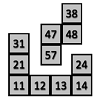100 Square Jigsaw

Age 5 to 7 Challenge Level:

Can you complete this jigsaw of the 100 square?Squares in Rectangles

Age 11 to 14 Challenge Level:

A 2 by 3 rectangle contains 8 squares and a 3 by 4 rectangle contains 20 squares. What size rectangle(s) contain(s) exactly 100 squares? Can you find them all?Coordinate Patterns

Age 11 to 14 Challenge Level:

Charlie and Alison have been drawing patterns on coordinate grids. Can you picture where the patterns lead?Age 11 to 14 Challenge Level:

Choose a couple of the sequences. Try to picture how to make the next, and the next, and the next... Can you describe your reasoning?More Number Pyramids

Age 11 to 14 Challenge Level:

When number pyramids have a sequence on the bottom layer, some interesting patterns emerge...Proof Sorter - Sum of an AP

Age 16 to 18 Challenge Level:

Use this interactivity to sort out the steps of the proof of the formula for the sum of an arithmetic series. The 'thermometer' will tell you how you are doingPrime AP

Age 16 to 18 Challenge Level:

What can you say about the common difference of an AP where every term is prime?Days and Dates

Age 11 to 14 Challenge Level:

Investigate how you can work out what day of the week your birthday will be on next year, and the year after...Domino Sequences

Age 5 to 7 Challenge Level:

Find the next two dominoes in these sequences.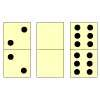Domino Number Patterns

Age 5 to 7 Challenge Level:

Can you work out the domino pieces which would go in the middle in each case to complete the pattern of these eight sets of 3 dominoes?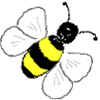Buzzy Bee

Age 5 to 7 Challenge Level:

Buzzy Bee was building a honeycomb. She decorated the honeycomb with a pattern using numbers. Can you discover Buzzy's pattern and fill in the empty cells for her?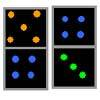Next Domino

Age 5 to 7 Challenge Level:

Which comes next in each pattern of dominoes?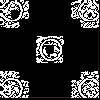Doplication

Age 7 to 11 Challenge Level:

We can arrange dots in a similar way to the 5 on a dice and they usually sit quite well into a rectangular shape. How many altogether in this 3 by 5? What happens for other sizes?Pocket Money

Age 11 to 14 Challenge Level:

Which of these pocket money systems would you rather have?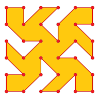Maxagon

Age 11 to 14 Challenge Level:

What's the greatest number of sides a polygon on a dotty grid could have?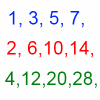Odds, Evens and More Evens

Age 11 to 14 Challenge Level:

Alison, Bernard and Charlie have been exploring sequences of odd and even numbers, which raise some intriguing questions...What Numbers Can We Make?

Age 11 to 14 Challenge Level:

Imagine we have four bags containing a large number of 1s, 4s, 7s and 10s. What numbers can we make?Charlie's Delightful Machine

Age 11 to 16 Challenge Level:

Here is a machine with four coloured lights. Can you develop a strategy to work out the rules controlling each light?A Little Light Thinking

Age 14 to 16 Challenge Level:

Here is a machine with four coloured lights. Can you make two lights switch on at once? Three lights? All four lights?Slick Summing

Age 14 to 16 Challenge Level:

Watch the video to see how Charlie works out the sum. Can you adapt his method?Speedy Summations

Age 16 to 18 Challenge Level:

Watch the video to see how to add together an arithmetic sequence of numbers efficiently.Prime Sequences

Age 16 to 18 Challenge Level:

This group tasks allows you to search for arithmetic progressions in the prime numbers. How many of the challenges will you discover for yourself?Mobile Numbers

Age 5 to 11 Challenge Level:

In this investigation, you are challenged to make mobile phone numbers which are easy to remember. What happens if you make a sequence adding 2 each time?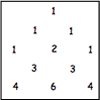Investigating Pascal's Triangle

Age 7 to 14 Challenge Level:

In this investigation, we look at Pascal's Triangle in a slightly different way - rotated and with the top line of ones taken off.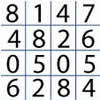Tables Without Tens

Age 7 to 11 Challenge Level:

Investigate and explain the patterns that you see from recording just the units digits of numbers in the times tables.Squares, Squares and More Squares

Age 11 to 14 Challenge Level:

Can you dissect a square into: 4, 7, 10, 13... other squares? 6, 9, 12, 15... other squares? 8, 11, 14... other squares?Polite Numbers

Age 16 to 18 Challenge Level:

A polite number can be written as the sum of two or more consecutive positive integers. Find the consecutive sums giving the polite numbers 544 and 424. What characterizes impolite numbers?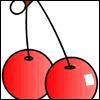Cherries Come in Twos

Age 7 to 11 Challenge Level:

Susie took cherries out of a bowl by following a certain pattern. How many cherries had there been in the bowl to start with if she was left with 14 single ones?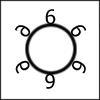Six in a Circle

Age 5 to 7 Challenge Level:

If there is a ring of six chairs and thirty children must either sit on a chair or stand behind one, how many children will be behind each chair?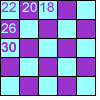Transformations Tables

Age 7 to 11 Challenge Level:

These grids are filled according to some rules - can you complete them?Red Balloons, Blue Balloons

Age 7 to 11 Challenge Level:

Katie and Will have some balloons. Will's balloon burst at exactly the same size as Katie's at the beginning of a puff. How many puffs had Will done before his balloon burst?Series Sums

Age 14 to 16 Challenge Level:

Let S1 = 1 , S2 = 2 + 3, S3 = 4 + 5 + 6 ,........ Calculate S17.Natural Sum

Age 14 to 16 Challenge Level:

The picture illustrates the sum 1 + 2 + 3 + 4 = (4 x 5)/2. Prove the general formula for the sum of the first n natural numbers and the formula for the sum of the cubes of the first n natural. . . .Summats Clear

Age 16 to 18 Challenge Level:

Find the sum, f(n), of the first n terms of the sequence: 0, 1, 1, 2, 2, 3, 3........p, p, p +1, p + 1,..... Prove that f(a + b) - f(a - b) = ab.Age 16 to 18 Challenge Level:

In y = ax +b when are a, -b/a, b in arithmetic progression. The polynomial y = ax^2 + bx + c has roots r1 and r2. Can a, r1, b, r2 and c be in arithmetic progression?Be Reasonable

Age 16 to 18 Challenge Level:

Prove that sqrt2, sqrt3 and sqrt5 cannot be terms of ANY arithmetic progression.Skip Counting

Age 5 to 7 Challenge Level:

Find the squares that Froggie skips onto to get to the pumpkin patch. She starts on 3 and finishes on 30, but she lands only on a square that has a number 3 more than the square she skips from.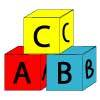Alphabet Blocks

Age 5 to 11 Challenge Level:

These alphabet bricks are painted in a special way. A is on one brick, B on two bricks, and so on. How many bricks will be painted by the time they have got to other letters of the alphabet?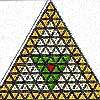The Great Tiling Count

Age 7 to 11 Challenge Level:

Compare the numbers of particular tiles in one or all of these three designs, inspired by the floor tiles of a church in Cambridge.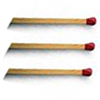Matchsticks

Age 7 to 11 Challenge Level:

Reasoning about the number of matches needed to build squares that share their sides.